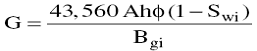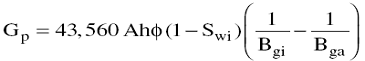# The Volumetric Method Assignment Help

## The Volumetric Method

The volumetric equation is useful in reserve work for estimating gas in place at any stage of depletion. During the development period before reservoir limits have been accurately defined, it is convenient to calculate gas in place per acre-foot of bulk reservoir rock. Multiplication of this unit figure by the best available estimate of bulk reservoir volume then gives gas in place for the lease, tract, or reservoir under consideration. Later in the life of the reservoir, when the reservoir volume is defined and Performance data are available, volumetric calculations provide valuable checks on gas in place estimates obtained from material balance methods.

The equation for calculating gas in place is:Where

G = gas in place, scf

A = area of reservoir, acres

h = average reservoir thickness, ft

f = porosity

Swi = water saturation, and

Bgi = gas formation volume factor, ft3/scf.

## The Volumetric Method Assignment Help By Online Tutoring and Guided Sessions from AssignmentHelp.Net

This equation can be applied at both initial and abandonment conditions in order to calculate the recoverable gas.

Gas produced = Initial gas - Remaining gas

OrWhere

Bga is evaluated at abandonment pressure. Application of the volumetric method assumes that the pore volume occupied by gas is constant. If water influx is occurring, A, h, and Sw will change.